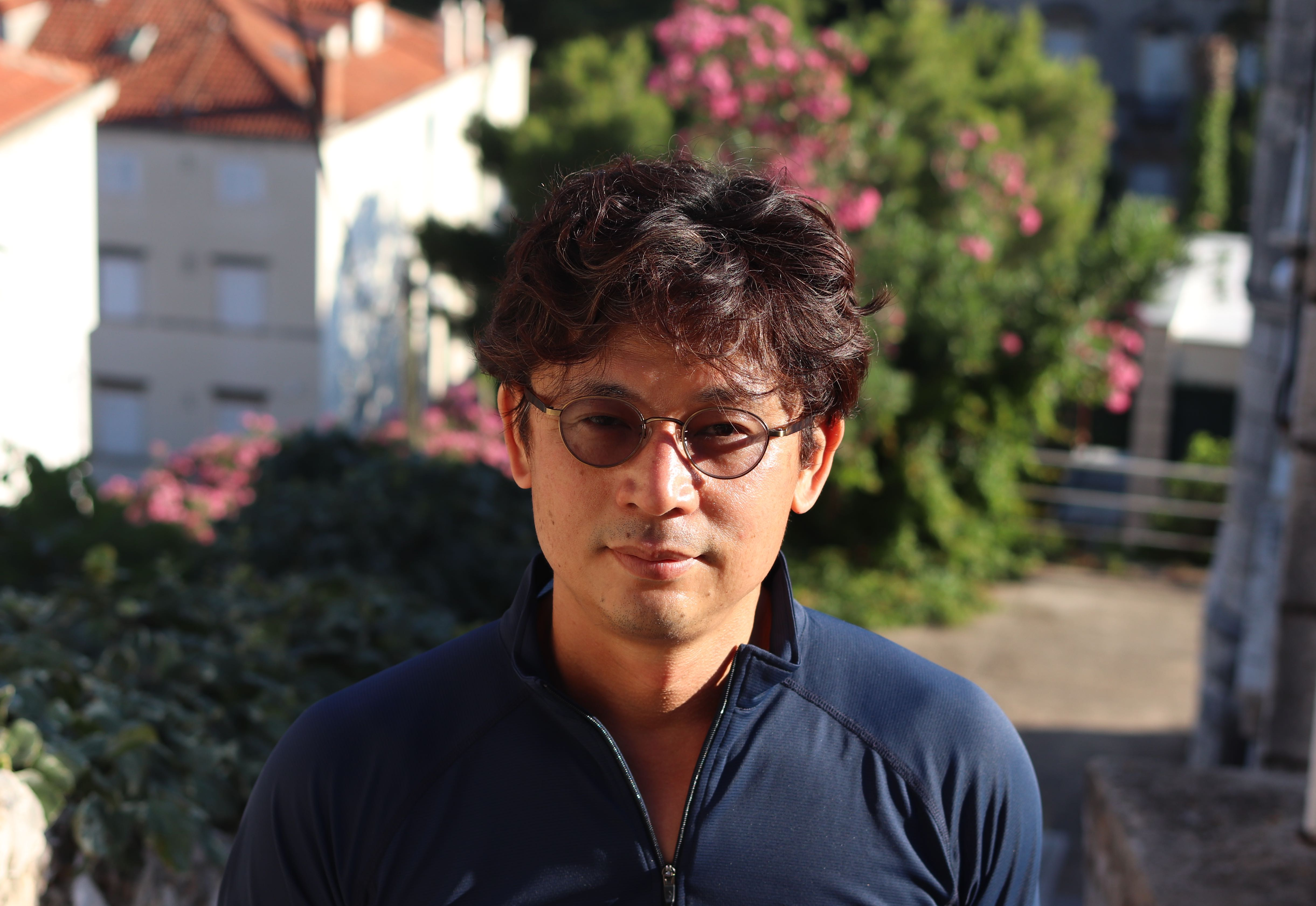# Tomoyuki ArakawaProfessor

Research Institute for Mathematical Sciences, Kyoto University

E-MAIL: arakawa at kurims.kyoto-u.ac.jp

Research Interest: Representation Theory, Vertex Algebras

Last Update： 13-Nov-2019

Curriculum Vitae (pdf)

Some videos of my talks

RIMS Representation Theory Seminar

Past Conferences

Some recent papers and preprints:

1. (with H. Yamada and H. Yamauchi) $\mathbb{Z}_k$-code vertex operator algebras, arXiv:1907.10216 [math.RT].
2. (with J. van Ekeren) Rationality and Fusion Rules of Exceptional W-Algebras, arXiv:1905.11473 [math.RT].
3. Chiral algebras of class $\mathcal{S}$ and Moore-Tachikawa symplectic varieties, arXiv:1811.01577 [math.RT].
4. (with E. Frenkel) Quantum Langlands duality of representations of W-algebras, Compos. Math. Volume 155, Issue 12, December 2019, 2235-2262.
5. (with A. Linshaw) Singular support of a vertex algebra and the arc space of its associated scheme. In: Gorelik M., Hinich V., Melnikov A. (eds) Representations and Nilpotent Orbits of Lie Algebraic Systems. Progress in Mathematics, vol 330. Birkhäuser, Cham.
6. (with A. Moreau) Arc spaces and chiral symplectic cores, arXiv:1802.06533 [math.RT], to appear in the special issue of Publ. Res. Inst. Math. in honor of Professor Masaki Kashiwara's 70th birthday.
7. (with T. Creutzig and A. Linshaw) W-algebras as coset vertex algebras, Invent. Math., October 2019, Volume 218, Issue 1, pp 145–195.
8. Representation theory of W-algebras and Higgs branch conjecture, Proc. Int. Cong. of Math. 2018 Rio de Janeiro, Vol. 1 (1261-1278).
9. Associated Varieties and Higgs Branches (A Survey), Contemp. Math. 711(2018), 37-44.
10. (with C. Jiang) Coset Vertex Operator Algebras and W-Algebras, Sci. China Math. 61 (2018), no. 2, 191–206. 17B69.
11. (with C.-H. Lam and H. Yamada) Parafermion vertex operator algebras and W-algebrasTrans. Amer. Math. Soc. 371 (2019), 4277-4301.
12. (with J. van Ekeren) Modularity of relatively rational vertex algebras and fusion rules of principal affine W-algebras, Comm. Math. Phys. (2019), 370 (1), 205--247.
13. (with K. Kawasetsu) Quasi-lisse vertex algebras and modular linear differential equations, In: V. G. Kac, V. L. Popov (eds.), Lie Groups, Geometry, and Representation TheoryA Tribute to the Life and Work of Bertram Kostant, Progr. Math., 326, Birkhauser, 2018.
14. (with A. Moreau) Joseph ideals and lisse minimal W-algebras, J. Inst. Math. Jussieu, 17 (2018), no. 2, 397–417.

Here is the complete list of publications.

RIMS